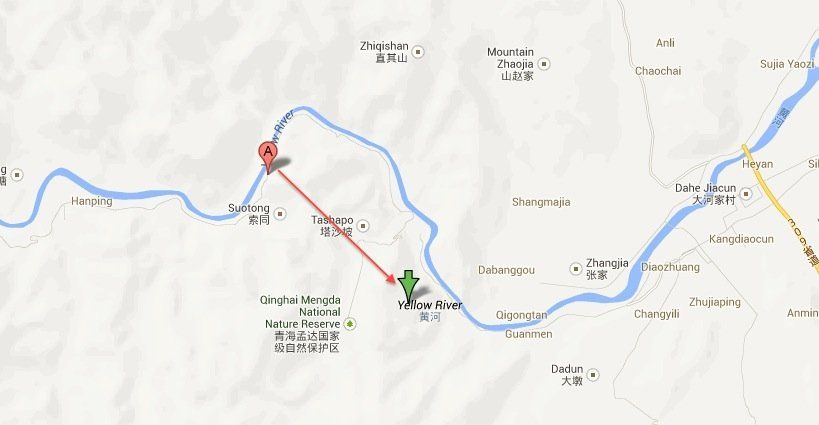# China GPS offset problem

## Jul 16, 2013 18:05 · 456 words · 3 minute read

Today I stumpled over a rather fascinating post on Sinosplice. It basically says that all maps in China are based on a different coordinate system than Western maps. As a result, ‘Western’ GPS-coordinates projected onto them will be off between 300 to 500m.I noticed this issue while playing Ingress in Shanghai. While walking along the Bund, I always ended up in the Pu-river. It seems that Google Maps has a correction-algorithm built in, while Ingress hasn’t. This still doesn’t help you while tagging photos or sharing your position with friends.

Luckily the conversion can be done in a couple lines of code. There are different versions around. I used the recommended C# version and put it into Python to use it more easily on non-Windows systems. All credit goes to the original anonymous author.

[cc lang=“python” width=“100%“tab_size=“4” lines=“40” noborder=“1” theme=“dawn”]

#!/usr/bin/env python

#Source: https://on4wp7.codeplex.com/SourceControl/changeset/view/21455#EvilTransform.cs
# NO WARRANTY OR GUARANTEE

import math

a = 6378245.0
ee = 0.00669342162296594323

def transformLat(x, y):
ret = -100.0 + 2.0 * x + 3.0 * y + 0.2 * y * y + 0.1 * x * y + 0.2 * math.sqrt(math.fabs(x));
ret += (20.0 * math.sin(6.0 * x * math.pi) + 20.0 * math.sin(2.0 * x * math.pi)) * 2.0 / 3.0;
ret += (20.0 * math.sin(y * math.pi) + 40.0 * math.sin(y / 3.0 * math.pi)) * 2.0 / 3.0;
ret += (160.0 * math.sin(y / 12.0 * math.pi) + 320 * math.sin(y * math.pi / 30.0)) * 2.0 / 3.0;
return ret;

def transformLon(x, y):
ret = 300.0 + x + 2.0 * y + 0.1 * x * x + 0.1 * x * y + 0.1 * math.sqrt(math.fabs(x));
ret += (20.0 * math.sin(6.0 * x * math.pi) + 20.0 * math.sin(2.0 * x * math.pi)) * 2.0 / 3.0;
ret += (20.0 * math.sin(x * math.pi) + 40.0 * math.sin(x / 3.0 * math.pi)) * 2.0 / 3.0;
ret += (150.0 * math.sin(x / 12.0 * math.pi) + 300.0 * math.sin(x / 30.0 * math.pi)) * 2.0 / 3.0;
return ret;

def transform (wgLat, wgLon):
dLat = transformLat(wgLon - 105.0, wgLat - 35.0);
dLon = transformLon(wgLon - 105.0, wgLat - 35.0);
radLat = wgLat / 180.0 * math.pi;